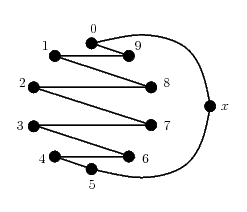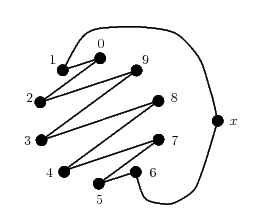# Internet Problem Solving Contest

## Solution to Problem I – Ideal Matches

Let's translate the problem statement into the graph theoretical terms. We are given a complete graph K2N and one (arbitrary) fixed perfect matching M in this graph. The goal is to split the remaining edges into 2N-2 perfect matchings such that each of these matchings together with M forms a Hamiltonian cycle in the graph.

This is known as a "semi-perfect 1-factorization". One possible method how to find such a factorization is given in the article Rastislav Kralovic and Richard Kralovic: On semi-perfect 1-factorizations. In A. Pelc and M. Raynal, editors, SIROCCO, volume 3499 of Lecture Notes in Computer Science, pages 216-230. Springer, 2005. You can find the full text of this article here.

Our solution uses the method described in the article. To construct the factorization in this way, we have to decompose KN* (the directed complete graph) into directed Hamiltonian cycles.

Here we may note that large Ns in the input file are all odd. For odd N there is a simple way how to decompose KN into (normal, not directed) Hamiltonian cycles:

The first cycle will look as follows:We will obtain the next cycles by rotating the circular widget (i.e., everything except the rightmost node). For example, the second cycle will look as follows:We then replace each Hamiltonian cycle by two directed ones and apply the construction from the article cited above.